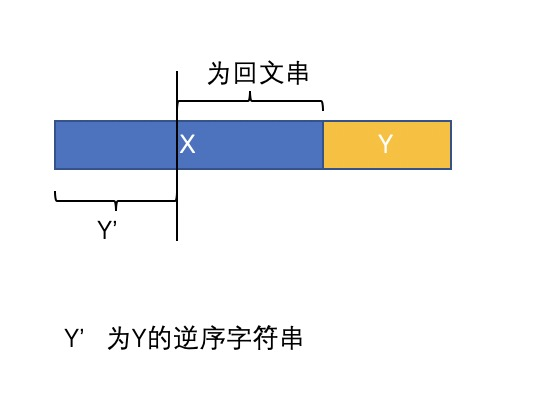# LeetCode - 回文串（序列）类总结（dp解法）

• 子串与子序列
（1）字符子串：指的是字符串中连续的n个字符，如abcdefg中，ab，cde，fg等都属于它的字串。
（2）字符子序列：指的是字符串中不一定连续但先后顺序一致的n个字符，即可以去掉字符串中的部分字符，但不可改变其前后顺序。如abcdefg中，acdg，bdf属于它的子序列，而bac，dbfg则不是，因为它们与字符串的字符顺序不一致。
(3) 公共子序列：如果序列C既是序列A的子序列，同时也是序列B的子序列，则称它为序列A和序列B的公共子序列。如对序列 1,3,5,4,2,6,8,7和序列 1,4,8,6,7,5 来说，序列1,8,7是它们的一个公共子序列。最长公共子序列（LCS）：就是A和B的公共子序列中长度最长的（包含元素最多的），最长公共子序列（LCS）也不一定唯一，但是长度一定。

class Solution {
public:
string longestPalindrome(string s) {
int length = s.size();
if (length == 0){
return s;
}
int maxCount = 0;
int left = 0;
int right = 0;
vector<vector<bool>> dp(length, vector<bool>(length,false));
for (int i = 0; i < length; i++)
{
for (int j = i; j >= 0; j--){
dp[i][j] = (s[i] == s[j]) && ((i - j < 3) || dp[i-1][j+1]);
if (dp[i][j] && (i - j + 1>maxCount))
{
maxCount = i - j + 1;
left = j;
right = i;
}
}
}
return s.substr(left, right-left+1);
}
};


class Solution {
public:
int countSubstrings(string s) {
int length = s.size();
if (length == 0){
return 0;
}
int count = 0;

vector<vector<bool>> dp(length, vector<bool>(length,false));
for (int i = 0; i < length; i++)
{
count++;
for (int j = i-1; j >= 0; j--){
dp[i][j] = (s[i] == s[j]) && ((i - j < 3) || dp[i-1][j+1]);
if (dp[i][j])
{
count ++;
}
}
}
return count;
}
};


class Solution {
public:
int longestPalindromeSubseq(string s) {
if(s.empty()) return 0;
int n=s.size();
vector<vector<int> > dp(n,vector<int>(n,0));
for(int j=0;j<n;j++){
dp[j][j]=1;
for(int i=j-1;i>=0;i--){
if(s[i]==s[j]) dp[i][j]=dp[i+1][j-1]+2;
else dp[i][j]=max(dp[i+1][j],dp[i][j-1]);
}
}
return dp[n-1];
}
};


1.当x.length()≥y.length()时, 字符串x的y.length()长度的前缀与y的逆序相等，且字符串x去除长度为y.length()的前缀后，余下的部分也是一个回文字符串。
2.当x.length() < y.length()时,与情况一正相反。如下图所示，要分析Y在X前，Y在X后的两种情况。class Solution {
public:
bool f(string& s,int left,int right){
while(left<right){
if(s[left++]!=s[right--]) return false;
}
return true;
}
vector<vector<int>> palindromePairs(vector<string>& words) {
unordered_map<string,int> m;
set<int> s;
int n=words.size();
for(int i=0;i<n;i++){
m[words[i]]=i;
s.insert(words[i].size());
}
vector<vector<int>> res;
for(int i=0;i<n;i++){
string tmp=words[i];
reverse(tmp.begin(),tmp.end());
if(m.count(tmp)&&m[tmp]!=i){
res.push_back({m[tmp],i});
}
int length=tmp.size();
for(auto it=s.begin();*it!=length;it++){
int d=*it;
if(f(tmp,0,length-d-1)&&m.count(tmp.substr(length-d))){
res.push_back({i,m[tmp.substr(length-d)]});
}
if(f(tmp,d,length-1)&&m.count(tmp.substr(0,d))){
res.push_back({m[tmp.substr(0,d)],i});
}
}
}
return res;
}
};


// 只有0个或1个字符出现奇数次，其余出现偶数次
class Solution {
public:
bool canPermutePalindrome(string s) {
unordered_map<char, int> m;
for(char c:s)
m[c]+=1;
int odd = 0;
for(auto it = m.begin(); it!=m.end();++it)
if(it->second % 2 == 1)
odd ++;
return (odd == 0) || (odd == 1);
}
};



   /*思路  如对于串 abcd 想要将其变为回文串
那么先把它逆序 然后放在前面 自然是回文了
abcd
dcba
dcbaabcd ->是回文
但是我们发现根本没必要放这么多在前面 因为abcd的前缀和dcab的后缀有重合(如a) 所以为了只添加最少的字符,我们在前方只需要添加不重复的即可
abcd
dcba
dcbabcd ->依然是回文
//为了添加的最少 我们就需要找到dcba的后缀和abcd的前缀重合的部分，且让重合部分最大即可
//故而联想到kmp算法，它的next数组就是用来求一个串的前缀和后缀相同的长度的最大值
//所以拼接起字符串 abcddcba 但是我们所求的前缀是不能超过中点的，因此用一个特殊字符隔开
//           即为 abcd#dcba 这样在匹配前后缀时，相同长度就一定不会超过#号了
//           这样问题就转化为了 求abcd#dcba的next数组 易知该串的前后缀相同时的最大长度为1
此时的最长相同前后缀即为a 和 a
所以把后半部分除去重叠的部分拼接到前半部分即可
答案就是  dcbabcd
大功告成!

*/
string shortestPalindrome(string s) {
string revs = s;//存s的逆序
int tn = s.size();//中点处,#前面的位置
reverse(revs.begin(),revs.end());
s = ' '+ s + '#' + revs;//让下标从1开始
int n = s.size()-1;//实际长度
vector<int> ne(n+1);//next数组
for(int i = 2, j = 0; i <= n; i++){//求next数组
while(j&&s[i]!=s[j+1]) j = ne[j];
if(s[i]==s[j+1]) j++;
ne[i] = j;
}
return s.substr(tn+2,tn-ne[n])+s.substr(1,tn);//后半部分除去重叠后缀+前半部分
}



©️2019 CSDN 皮肤主题: 大白 设计师: CSDN官方博客